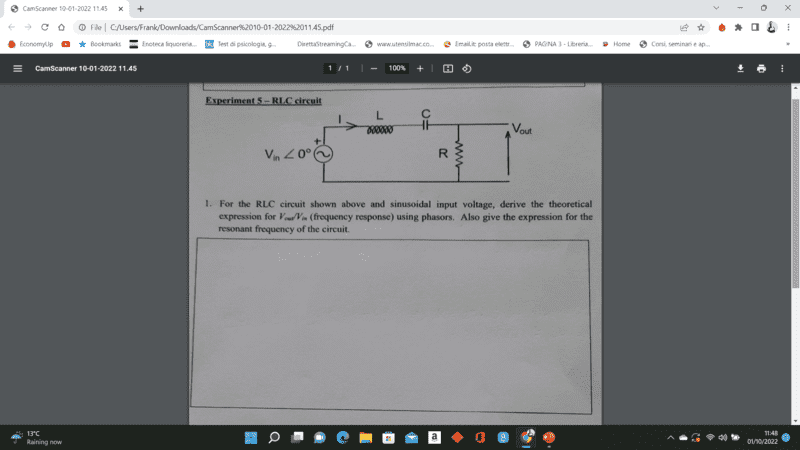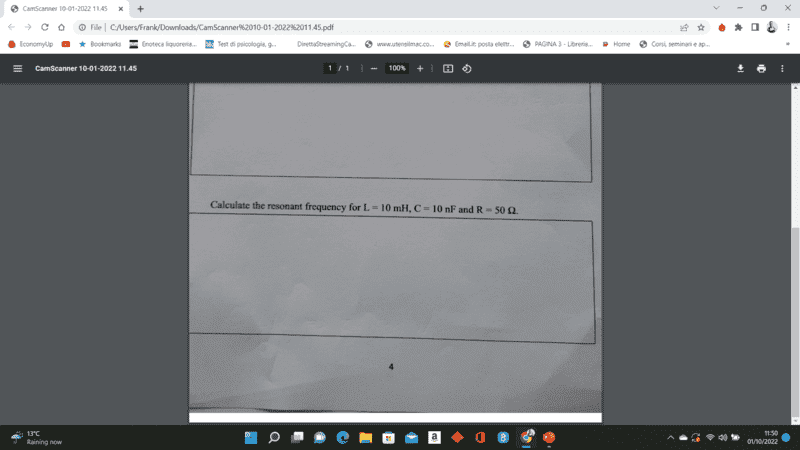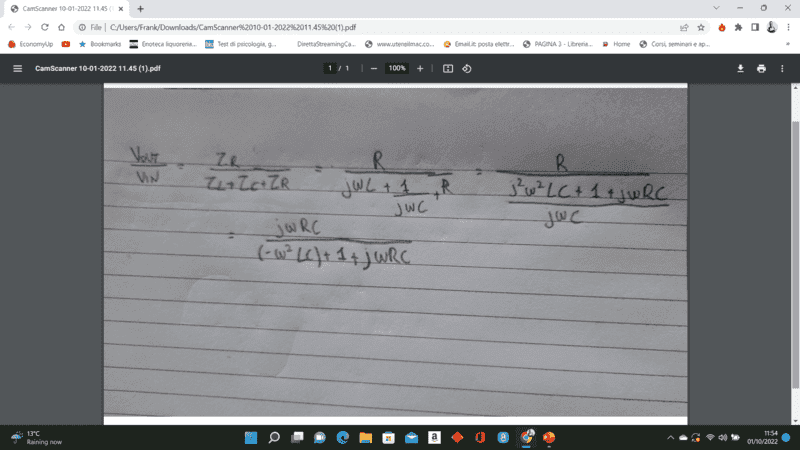# RLC circuit -- Phasor Transfer Function calculation

• Engineering
• Franklie001
In summary, the circuit will have a maximum amplitude at resonance. Work out that expression and ##\omega_{res}## can be found.

#### Franklie001

Homework Statement
For the RLC circuit shown above and sinusoidal input voltage, derive the theoretical expression for Vout/Vin (frequency response) using phasors. Also, give the expression for the resonant frequency of the circuit.
Calculate the resonant frequency for L=10mH, C=10nF, R=50 ohm
Relevant Equations
Vout/Vin
Good morning,

I need some help solving those two question. I've attached my attempted solution below. Could i solve the transfer function any further?#### Attachments

• CamScanner 10-01-2022 11.45.pdf
134.3 KB · Views: 69
•DaveE
Hi,

From the "using phasors" in the problem statement, I suspect you are asked to derive an expression that clearly shows amplitude (i.e. ## \left | {V_{R}\over V_{in}} \right | ## ) and phase difference.

##\ ##

Frequency response analysis in Finite Element Analysis (FEA) is used to calculate the steady-state response due to a sinusoidal load applied to a structure at a single frequency. It is a specialized type of transient response analysis that is extremely efficient to solve a very specific type of model.
I think in RLC circuit the equation has to be:
VL+VR+VC=f(t) if no load is out. f(t)=F1sin(ωt)+F2cos (ωt)={F}e^iωt
Then the derivate will be Ld2i/dt2+Rdi/dt+i/C=-ω^2{F}e^iωt

thanks for your response. Could i ask why you derivate the expression? I'm sure that question asks to solve for the frequency response Vout/Vin of the circuit and its resonant frequency.
I had an attempt at it but unsure how to derive the expression for the resonant frequency?
Also, in the second question, I've been given a value of the resistor to calculate the resonant frequency. Could i ask why? Isn't the resonant frequency condition is XL = XC ?

Thanks again

The circuit will have a maximum ## \left | {V_{R}\over V_{in}} \right | ## at resonance.
Work out that expression and ##\omega_{res}## can be found. Rather easily.

Franklie001 said:
Perhaps to confuse you ?

##\ ##

BvU said:
The circuit will have a maximum ## \left | {V_{R}\over V_{in}} \right | ## at resonance.
Work out that expression and ##\omega_{res}## can be found. Rather easily.

Perhaps to confuse you ?

##\ ##
Hi, thank again for your help. I really appreciate it
Just to clarify ..isn't Vr/Vin what i was trying to find?
Also, It looks to me to be a bandpass filter from the expression found previously.
Also, how would you simply the expression for Vout/Vin for transfer function?

Franklie001 said:
Isn't the resonant frequency condition is XL = XC ?
Yes, this is the common answer and it works well for highly resonant systems (i.e. high Q, low damping). The real answer does depend a bit on the losses (resistance), but I'm not sure they really intend for you to account for that. For the values given the difference is quite insignificant.

https://en.wikipedia.org/wiki/RLC_circuit#Overdamped_response

See-for instance:

Franklie001 said:
Just to clarify ..isn't Vr/Vin what i was trying to find?
Yes. From the "using phasors" in the problem statement I conclude your textbook (or notes) should have something along those lines. Also, ##V_{in}## is given as a phasor: ##V_{in}\ \angle \ 0^\circ##.
So an answer along these lines (or any suitable other) seems indicated.

Franklie001 said:
Also, It looks to me to be a bandpass filter from the expression found previously.
Yes. The frequency characteristic ##\left | {V_{R}\over V_{in}} \right |## as a function of ##\omega## shows a peak at the resonance frequency.

Franklie001 said:
Also, how would you simply the expression for Vout/Vin for transfer function?
That, for me, is the essence of the job (your job) at hand !And your link in #8 is not very useful in this context.

Your work in post #1 is more or less the start of an algebraic analysis (an approach that I strongly support; much better in an introductory stage (correct me if I am wrong, but I estimate you are still in that stage).

So you have $${V_{R}\over V_{in}} = {R\over R + j\left ( \omega L - \displaystyle {1\over \omega C} \right ) }$$
How can you write this in the form the exercise seems to prefer: ##A\ \angle\ \phi## ? ( Note that I tried to help a little bit by writing the numerator in this way)

##\ ##

•SammyS
DaveE said:
Yes, this is the common answer and it works well for highly resonant systems (i.e. high Q, low damping). The real answer does depend a bit on the losses (resistance), but I'm not sure they really intend for you to account for that. For the values given the difference is quite insignificant.

https://en.wikipedia.org/wiki/RLC_circuit#Overdamped_response
Thank you for the help I ll read this as soon as I can